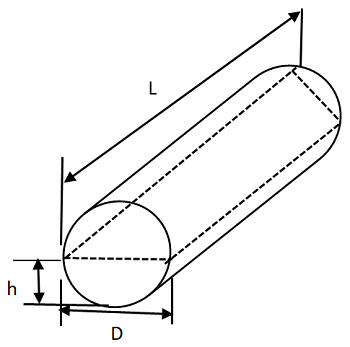Related Resources: calculators

### Horizontal Tank Draining Time Formulas and Calculator

Draining Time for Horizontal Tank Formulas and CalculatorHorizontal Tank Drain From Bottom

t = ( 0.166 L [ D1.5 - (D - h)1.5 ] ) / ( Cd An )

Where:

t = time to empty in seconds,
D = diameter of the cylinder, ft,
L = Length, ft
h = height of liquid in the cylinder, ft,
Cd = Discharge coefficient,
0.61 for sharp-edged orifice
0.8 for short flush mounted nozzle
0.98 for rounded orifice
An = Cross-sectional area of drain opening (orifice / nozzle) based on ID of orifice / nozzle, ft2 ,
An = π/4 ( d / 12)2

References:

Reference: Section 5, Chemical & Process Plant Engineering
Standard Handbook of Engineering Calculations, 4th Edition, Tyler G. Hicks

Related:

Draining Time for Vertical Tank Formulas and Calculator